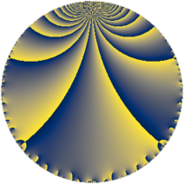Properties

 Label 36.3.gLevel $36$ Weight $3$ Character orbit 36.g Rep. character $\chi_{36}(5,\cdot)$ Character field $\Q(\zeta_{6})$ Dimension $4$ Newform subspaces $1$ Sturm bound $18$ Trace bound $0$

Related objects

Defining parameters

 Level: $$N$$ $$=$$ $$36 = 2^{2} \cdot 3^{2}$$ Weight: $$k$$ $$=$$ $$3$$ Character orbit: $$[\chi]$$ $$=$$ 36.g (of order $$6$$ and degree $$2$$) Character conductor: $$\operatorname{cond}(\chi)$$ $$=$$ $$9$$ Character field: $$\Q(\zeta_{6})$$ Newform subspaces: $$1$$ Sturm bound: $$18$$ Trace bound: $$0$$

Dimensions

The following table gives the dimensions of various subspaces of $$M_{3}(36, [\chi])$$.

Total New Old
Modular forms 30 4 26
Cusp forms 18 4 14
Eisenstein series 12 0 12

Trace form

 $$4 q + 3 q^{3} + 9 q^{5} - q^{7} - 15 q^{9} + O(q^{10})$$ $$4 q + 3 q^{3} + 9 q^{5} - q^{7} - 15 q^{9} - 36 q^{11} + 5 q^{13} - 45 q^{15} + 2 q^{19} + 99 q^{21} + 99 q^{23} + 13 q^{25} - 63 q^{29} - 7 q^{31} - 36 q^{33} - 64 q^{37} + 57 q^{39} - 18 q^{41} - 46 q^{43} - 99 q^{45} - 81 q^{47} - 51 q^{49} - 27 q^{51} + 90 q^{55} + 51 q^{57} + 126 q^{59} + 41 q^{61} + 141 q^{63} + 171 q^{65} + 116 q^{67} + 99 q^{69} + 86 q^{73} - 297 q^{75} - 279 q^{77} + 83 q^{79} - 63 q^{81} - 81 q^{83} + 18 q^{85} - 63 q^{87} - 302 q^{91} - 159 q^{93} - 144 q^{95} - 196 q^{97} + 171 q^{99} + O(q^{100})$$

Decomposition of $$S_{3}^{\mathrm{new}}(36, [\chi])$$ into newform subspaces

Label Dim. $$A$$ Field CM Traces $q$-expansion
$a_{2}$ $a_{3}$ $a_{5}$ $a_{7}$
36.3.g.a $4$ $0.981$ $$\Q(\sqrt{-3}, \sqrt{-11})$$ None $$0$$ $$3$$ $$9$$ $$-1$$ $$q+(1-\beta _{1}+\beta _{3})q^{3}+(2-2\beta _{1}-2\beta _{2}+\cdots)q^{5}+\cdots$$

Decomposition of $$S_{3}^{\mathrm{old}}(36, [\chi])$$ into lower level spaces

$$S_{3}^{\mathrm{old}}(36, [\chi]) \cong$$ $$S_{3}^{\mathrm{new}}(9, [\chi])$$$$^{\oplus 3}$$$$\oplus$$$$S_{3}^{\mathrm{new}}(18, [\chi])$$$$^{\oplus 2}$$